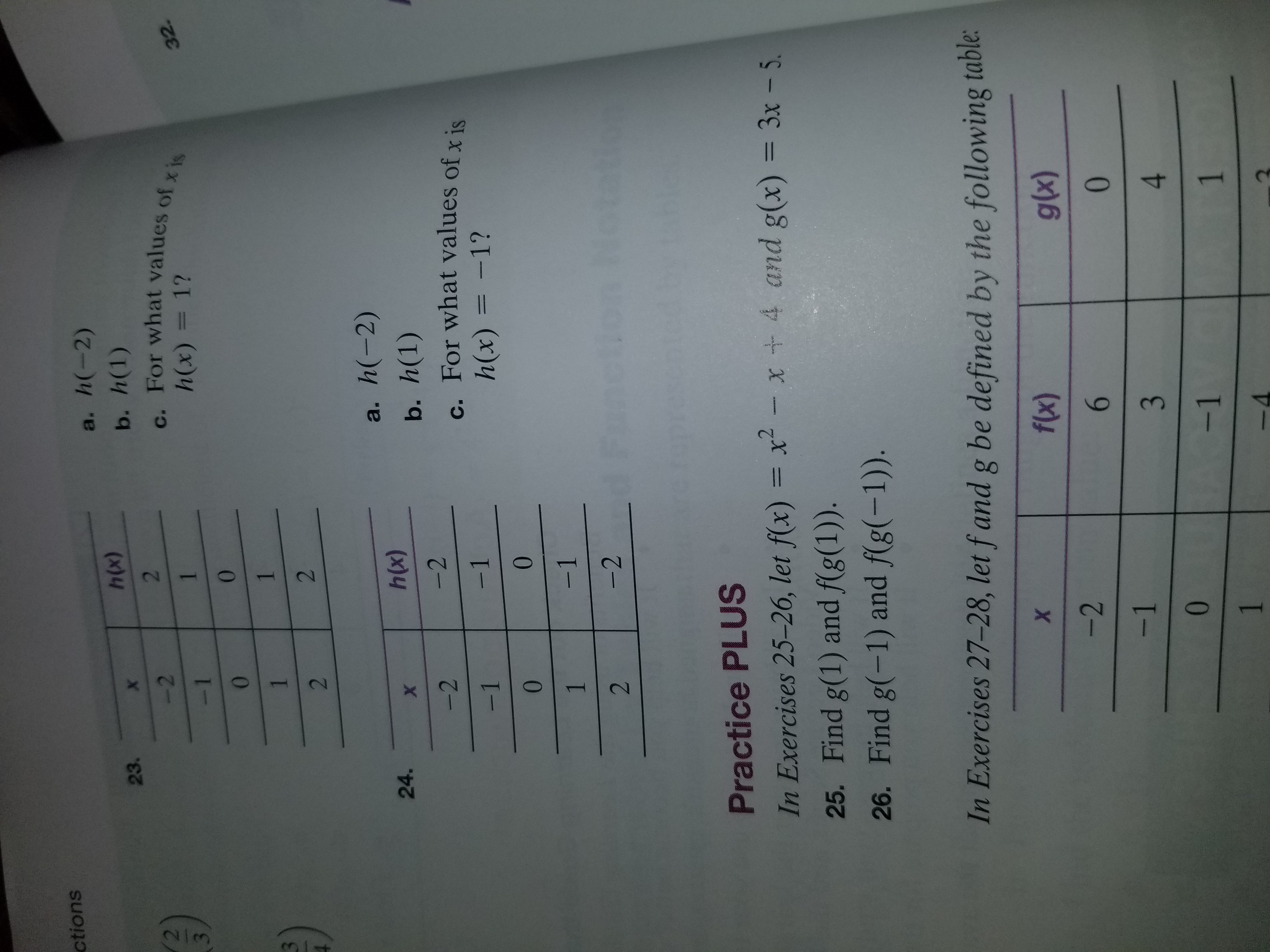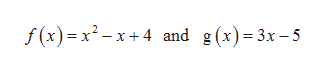ctionsh(-2)a.h(x)b. h(1)c. For what values of x ish(x) = 1?23.X2231-132.001122h(-2)a.h(x)24.Xb. h(1)c. For what values of x ish(x) =-1?-2-2-1-100-11-22Practice PLUSIn Exercises 25-26, let f(x) = x- x+4 and g(x) = 3x - 5.25. Find g(1) and f(g(1)).26. Find g(-1) and f(g(-1)).In Exercises 27-28, let fand g be defined by the following tableXf(x)glx)-20-1340-111

Question

The question is number 25. Find g(1) and f(g(1)) to the attached photo.

F(x)=x2-x+4

G(x)=3x-5

The answers are -2 and 10. I am having issues showing my work and reaching that conclusion.

Thank youhelp_outlineImage Transcriptionclosections h(-2) a. h(x) b. h(1) c. For what values of x is h(x) = 1? 23. X 2 2 3 1 -1 32. 0 0 1 1 2 2 h(-2) a. h(x) 24. X b. h(1) c. For what values of x is h(x) =-1? -2 -2 -1 -1 0 0 -1 1 -2 2 Practice PLUS In Exercises 25-26, let f(x) = x- x+4 and g(x) = 3x - 5. 25. Find g(1) and f(g(1)). 26. Find g(-1) and f(g(-1)). In Exercises 27-28, let fand g be defined by the following table X f(x) glx) -2 0 -1 3 4 0 -1 1 1 fullscreen
Step 1

The given functions are,help_outlineImage Transcriptionclosef (x) x2x4 and g (x) 3x-5 fullscreen
Step 2

The function g with the x value as 1 is...

Want to see the full answer?

See Solution

Want to see this answer and more?

Our solutions are written by experts, many with advanced degrees, and available 24/7

See Solution
Tagged in

Algebra